# Java Sketchpad Trouble Shooting

Converting a Sketchpad file to Java is much more convenient now that it is a built-in function. Just go to the file menu and Save As HTML. If the file is being saved to the folder that contains the jsp folder, it will be viewed on your default browser. If you are preparing something for web publication, it is advisable to test it in Java several times while drawing. Not all of the Sketchpad constructions can be converted to Java.

Java Sketchpad is still under development, so we can expect it to get better. For now, I have compiled a list (incomplete, no doubt) of things that do not work, along with some suggested ways of working around the problems. I look forward to deleting these items as they are fixed. There may be a few other problems with converting files that were created in GSP3. Those will not be addressed here.

Parametric Coloring - This feature is not supported.

Work-around: Some of the simpler applications of parametric coloring may be simulated with conditional objects. Take for example a line segment that is blue when a certain value, v, is positive, and red when it is negative. Calculate sqrt(sgn(v)). This will equal one when v is positive and will be undefined when v is negative. Mark a center point somewhere and dilate the line segment using the calculation as the dilation ratio. Color the image blue. This blue image will appear only if v is greater than zero. For the red image, do the same thing using the calculation sqrt(sgn(0-v)).

Iterated Objects - Iterated objects are not supported at all. This is not so surprising since, like parametric coloring, it is a new and rather complicated feature.

Work-around: Iterated constructions are repeated constructions. Some of them can be effected by taking more time and doing the whole constuction. It may be possible to vary the depth by having the objects depend on certain conditional objects.

Labels - Labels appear only on points, and the labels' styles cannot be changed.

No work-around

Angle Bisector - Angle bisectors are not supported.

Work-around: Normally I like to avoid measurements, but I have found no geometric construction that would work for all special cases, including 0ş and 180ş. This measurement and rotation method does.

 Start with angle ABC.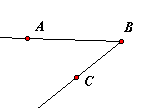Measure it.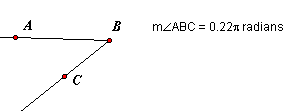Calculate one-half of the angle. Select the calculation and Mark Angle. Select point B and Mark Center.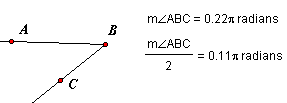Select point A and Rotate By Marked Angle. Construct ray (or line) BA'.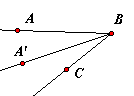Arcs - Arcs are not supported, no matter how they were constructed.

Work-around: There really is no adequate way to work around this omission unless all you need is something that looks like an arc. The arc can be supplanted with a locus. It may look right, but the locus does not have the same properties as the arc. It is not possible to construct the intersection of a locus and another object. A point may be attached to a locus in GSP, but that point and its descendents will not appear in the Java sketch. The method below is a cosmetic solution. It will work even for a major arc, but it collapses when the arc nears 360ş.

 Begin with an arc.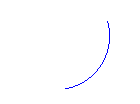Construct the circle that includes the arc, and construct the chord that joins the endpoints of the arc.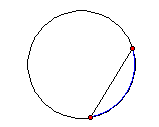Construct the perpendicular bisector of the chord. Let P be the point where it intersects the circle but not the arc. Hide the arc.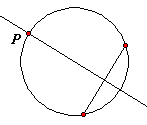Hide the perpendicular bisector. Construct point Q on the chord. Construct ray PQ. Let R be the point where the ray intersects the circle. Select points Q and R, and construct a locus.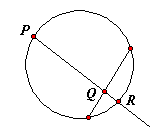Hide everything but the locus. See those tiny little squares at the end? I don't like them either, but they will not show up on the Java sketch. No matter what the line width setting is for a locus, it will appear thin in Java.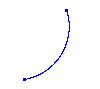Circle By Center+Radius - This can be used only if the center point and a line segment are selected. Do not use it with a measurement or a calculation. It will cause problems with the browser, even if the offending construction is hidden.

Work-around: Mark the measurement or calculation. Use that marked distance to do a polar translation on the center point. Then Construct Circle By Center+Point.

Dilate By Fixed Ratio - This method of dilation will not work on circles. Using a marked ratio is not a problem.

Work-around: Dilate the center and a point on the circle, and Construct Circle By Center+Point.

Translation (Polar) At Marked Angle - It is not possible to select three points, mark the angle, and use that marked angle for a polar translation. However, it is possible to mark an angle measurement or calculation, and use that.

Work-around: Measure the angle, mark the measurement, and use that in the translation.

Translation (Rectangular) At Marked Distance - A marked distance cannot be used in a rectangular translation. It will work in a polar translation.

Work-around: Do two polar translations, one at 0ş and one at 90ş.

Distance Units - Distances appear only in pixel units.

Work-around: The calculator can be used to strip each distance measurement of its units. Suppose that the desired unit is centimeters. Divide each distance by 1 cm. The result of the calculation will have no dimension, but it will have the correct numerical value, both in Sketchpad and in Java. The text tool can be used to place a unit label next to the number. The calculation and the label will stay together on the browser screen because these objects cannot be dragged.

Calculation - Java Sketchpad recognizes the minus sign only as a subtraction operator. It cannot be used as an opposite (negative) sign. Any calculations that use it this way will work in GSP, but they and their descendents will not appear in the Java sketch.

Work-around: Simply use a zero in front of it.

 This will not work. But this will. -p 0-p -5*m (0-5)*m

Measured Coordinates - From the Measure menu, the abscissa (x) or the ordinate (y) of any point can be measured, but those coordinates will not be recognized when the sketch is converted to Java.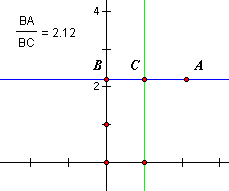Work-around: Let A be the point whose coordinates we want. From the Graph menu, show the axes and select a rectangular grid. Unit points will be displayed on both the x- and y-axes. Construct a line through the x-unit and parallel to the y-axis. This is the green line in the picture. Construct a line through A and parallel to the x-axis, the blue line. Construct intersection points B and C as shown. In order, select points B, C, A. Measure Ratio. The ratio is the x-coordinate of point A. The y-coordinate is measured by the same method, using the unit point on the y-axis. The measurements have no units and they will give correct values even if the scales of the axes are changed.

Plotted Points - Distance measurements are selected and Plot As (x,y) is executed from the Graph menu, but the point may be misplotted after the Java conversion.

Work-around: This is happening because the distance is being converted to pixel units. The easy solution is to go into Preferences and change all of the distance units to pixels, but that may be undesirable for some reason. A better way around the problem is to strip the units from the measurements before the point is plotted. For example, if a distance measurement is in inches, use the calculator to divide that measurement by 1 inch. Use the calculation in place of the measurement to plot the point.

Last update: November 27, 2006 ... Paul Kunkel whistling@whistleralley.com
For email to reach me, the word geometry must appear in the body of the message.